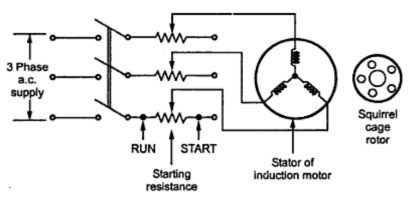### Stator Resistance Starter

In order to apply the reduced voltage to the stator of the induction motor, three resistances are are added in series with each phase of the stator winding. Initially the resistances are kept maximum in the circuit. Due to its large voltage gets dropped across the resistances. Hence a reduced voltage gets applied to the stator which reduces the high starting current. The schematic diagram showing stator resistances is shown in the Fig..1.Fig. 1 Stator Resistance starter

When the motor starts running, the resistances are gradually cut-off from the stator circuit. When the resistances are entirely removed from the stator circuit i.e. rheostats in RUN position then rated voltage gets applied to the stator. Motor runs with normal speed.
The starter is simple in construction and cheap. It can be used for both star and delta connected stator. But there are large power losses due to resistances. Also the starting torque of the motor reduces due to reduced voltage applied to the stator.
1.1 Relation between Tst and TF.L.
We know, P2 = T x ωs
where T is torque produced and P2 is the rotor input at Ns.
...                   T α  P2
But          P2 = Pc/s    where Pc = Total copper loss
= (3I2r2R2)/s
...                       T  α   I2r2/s
But rotor current I2r and stator current are related to each other through transformer action.
...                       T  α  I12 /s      where I= Stator current
At start,        s = 1,    T = Tst    and I= Ist
...                        Tst   α    Ist2                                                ........(1)
When stator resistance starter is used, the factory by which stator voltage reduces is say x < 1. The starting current is proportional to to this factor x. So if Isc is the normal current drawn under full rated voltage condition at start then,
Ist = x Isc                                                         ............(2)
...                        Tst  α  (xIsc)2                                                      ..........(3)
But              TF.L.  α  (IF.L.)2/sf    where sf  = Full load slip          ........(4)
Note : As x < 1, it can be seen that the starting torque reduces by the fraction x2 due to the stator resistance starter.

1.